# Hansen-Goos hard sphere equation of state

The Hansen-Goos hard sphere equation of state is given by  (Eq. 5):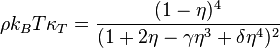$\rho k_B T \kappa_T = \frac{(1-\eta)^4}{(1+2\eta -\gamma \eta^3 + \delta \eta^4)^2}$

where (Eq. 7)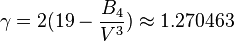$\gamma = 2(19- \frac{B_4}{V^3}) \approx 1.270463$

and (Eq. 8)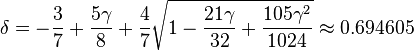$\delta = - \frac{3}{7} + \frac{5\gamma}{8} +\frac{4}{7} \sqrt{1- \frac{21 \gamma}{32} + \frac{105\gamma^2}{1024}} \approx 0.694605$

and where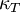$\kappa_T$ is the isothermal compressibility,$\rho$ is the number density and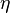$\eta$ is the packing fraction.